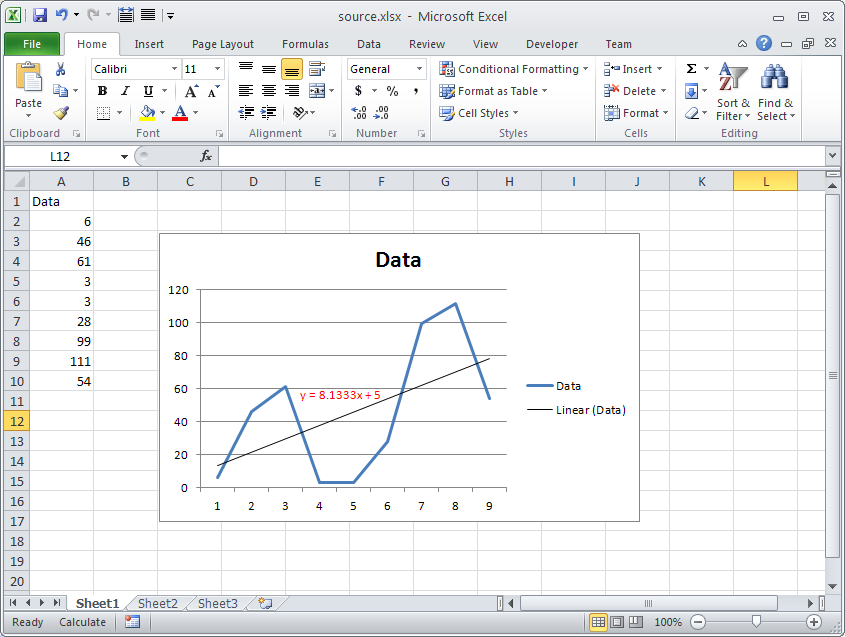# Get Equation Text of Chart Trendline

Contents
[ ]

## Example

The following screenshot shows the Chart with a Trendline and its Equation Text is shown in Red color. We will retrieve this text using the Trendline.getDataLabels().getText() property in the following sample code.### Output generated by the sample code

This is the console output of the above sample code.

``Equation Text: y = 8.1333x + 5``